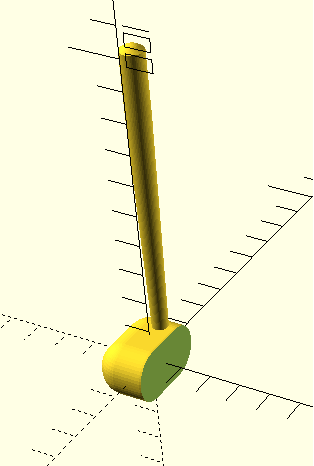So this week in OpenSCAD, I decided to do my best attempt at  a basic golf club since I was feeling sporty (no sports due to corona :p ). The code below is entirely my own.

My design process was very disorganized (organized chaos). I started making no particular part of this club in any order. I simply laid out all the frame work of what I had in mind. In other words, I knew I wanted to use the minkowski for the club head. I originally used a cylinder and then later replaced it with a sphere. I also tried to experiment with the rotate command here but I ended up scrapping it.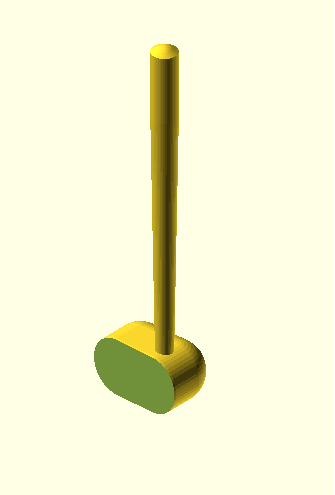When it comes to the details of my design, I’ve used two slightly offset cylinders hulled together to make the handle, as well as an offset sphere to give it a rounded top. The last piece was an arbitrarily large rectangle that I used to make the club face (difference command). None of the numbers have any significance other than what I thought made it look as good as possible.

I apologize ahead of time if my code is messy or confusing, I am not the best coder!
```\$fn=50;
difference() {
minkowski()
{
cube([8,12,1], center = true);
rotate(a=[0,0,0])
{sphere(8);
}
}
translate([15,0,0])
{
cube([20,40,50], center = true);
}
}
hull(){
translate([0,6,8])
{
cylinder(r=2.5,h=5);

}
translate([0,6,80])
{
cylinder(r=3.7,h=20);
}

translate([0,6,98])
{
sphere(3.7);
}
}
```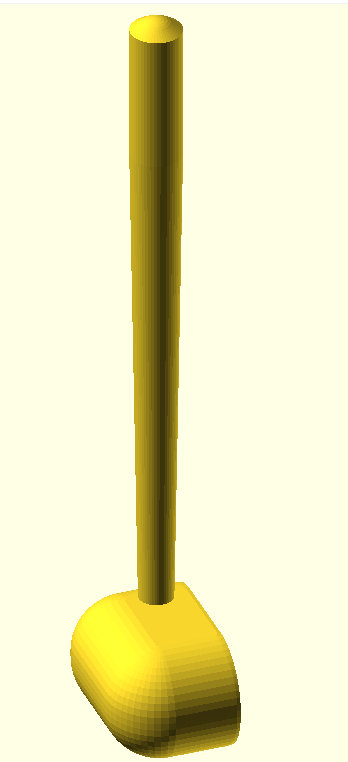This week I chose to design a basic Christmas ornament, however, I set a challenge for myself to create the spherical part of the ornament using a for-loop (which I learned over our meeting). In addition to the ornament ball, I added a hook. To make the hook I used the rotate extrude command, which I learned from the OpenSCAD User Manual. Some things that I don’t like about this design however, was that I was unable to figure out how to make a perfect circle using the rotating for-loop, and thus the bottom of the ornament looks very strange. Second, I was unable to center both the ornament and the cylindrical part of the hook so I translated and rotated until I thought the hook fit in the hole at the top of the ornament.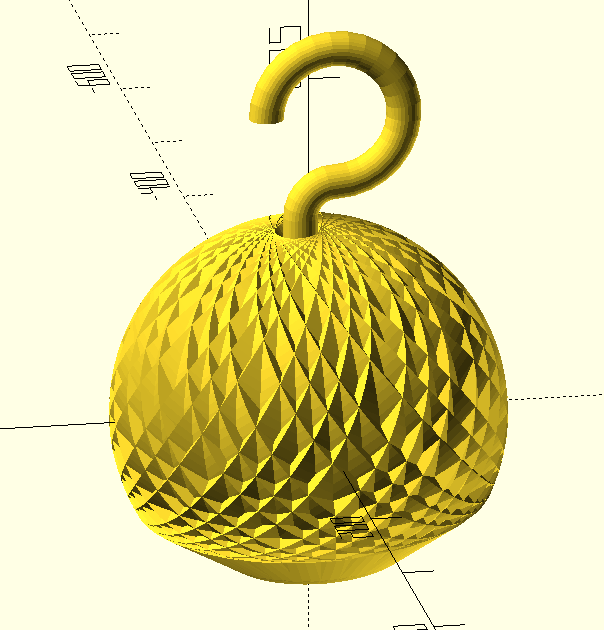Here is my code:

```//HOOK

translate(,[-14,8,120]){
rotate(90,[1,0,0]){
translate([0,60,0])
rotate_extrude(angle=270, convexity=10)
translate([40, 0]) circle(10);
rotate_extrude(angle=90, convexity=10)
translate([20, 0]) circle(10);
translate([20,0,0])
rotate([90,0,0]) cylinder(r=10,h=80);
}
}

//ORNAMENT
number_of_prisms = 30;

rotate(50,[1,0,0]){
rotate(40,[0,0,1]){
Crazy_Sphere (35);
}
}

module Prism(center = true){
translate([-10/2,0,0]){
rotate(90,[0,1,0]){
linear_extrude(60){
circle(100,\$fn=120);
}
}
}
}

module Crazy_Sphere(rotation_multiplier){

for (i=[0:number_of_prisms]){
rotate(rotation_multiplier*i, [1,1,1]){
Prism();
}
}
}```

So for my iteration goals I had two things in mind. Fix the club head to reflect more of a “driver” club head, and second tighten up and organize the code a bit more since my first design was rather messy. Part of the reason why I chose to iterate this design was I set out to make something realistic and my initial design was far from that.

I started by completely deleting the section of code dedicated for the head of the club and chose to organize the club into two parts, the club head and the pole. I then did some basic research on a driver head so I knew what shape to mimic.Photo Credits

I browsed the OpenSCAD manual (–very useful, would recommend) to see if there was any code that would help adapt my shape. I stumbled upon the resize tool and after some playing around with the code, this is what I came up with.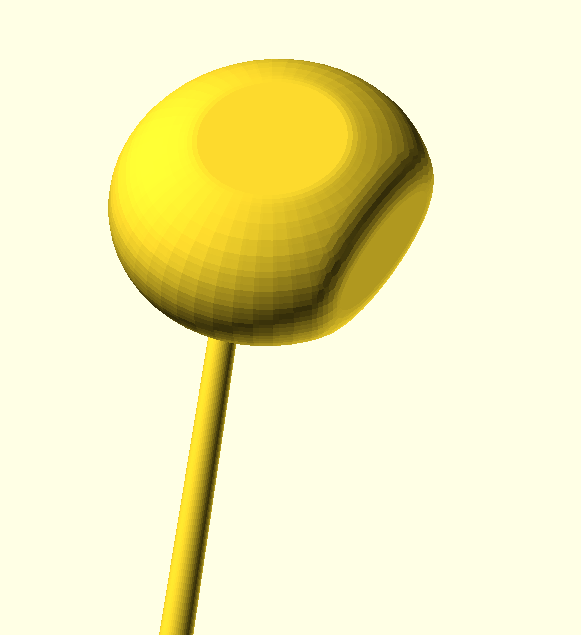Overall, I am fairly satisfied with the shape of the club head, however, I am not satisfied with how the club head and the pole connect (I am unsure on how to make this connection smoother). I think if I spent more time with this and played around more with the code, I would be able to make a more finished product, however, I think that process would require extra hours reading more of the manual which I simply did not have time for.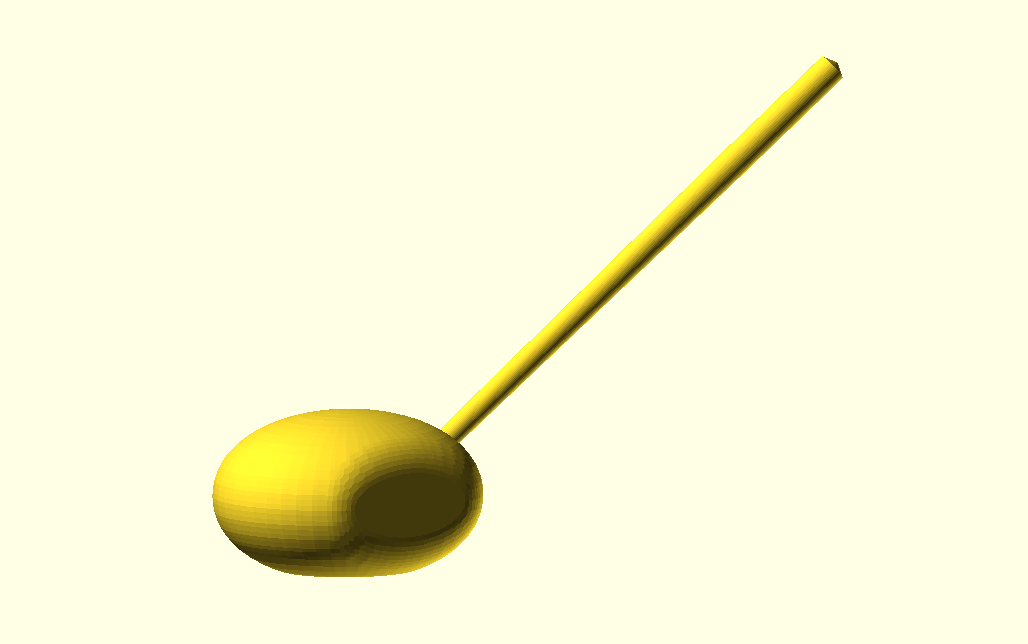And last, here is my code (completely redone from the first version):

```\$fn=50;

minkowski() {
difference(){
minkowski() {
difference(){
resize(newsize=[28,30,14]) sphere(r=10);
translate([30,0,0])
cube(40, center = true);
}
sphere(1);
}
translate([0,0,-26.5])
cube(40, center = true);
}
sphere(5);
}

////Final Pole

rotate([-45,20,0]){

///initial pole shape
hull(){
translate([0,6,8]){
cylinder(r=1.7,h=5);
}
translate([0,6,80]){
cylinder(r=2.3,h=20);
}

translate([0,6,100]){
sphere(1);
}
}
}```Photo Credits

My goals for this project is to create randomly generated tree stumps, of varying heights, and eventually have randomly generated roots. My goal for this week was to get the initial base design of the stump, incorporate the height element as well as generate the hole. However, I have had limited success. Currently, I have successfully generated the random aspect of the hole in the stump as seen below, however, I can’t seem to figure out how to vary the height. (note: the hole is not a simple sphere, it is a complex shape)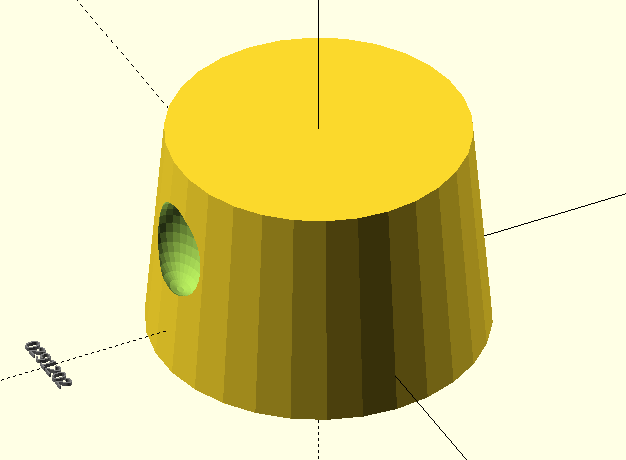Here is my code (currently set height at 60 since setting it equal to subrandom1 doesn’t yield successful results even after limiting to 1 random number):

```/////Goals for stump: Variable height or radius, varrying existance of roots/ hole on side

///test values
changing_height = 60;

////Stump

difference() {
translate([0, 0, 20]){
cylinder(h = changing_height , r1 = 45, r2 = 40, center = true);}
for (i=[0:10-1]){
if (subrandom2[i]==true) //stump hole existing?
translate([0, -40, 25])
resize(newsize=[24,16,26]) sphere(r=10); ////This is the hole shape!
}
}

///////COPY PASTE SEED GENERATION INFO/////////////

style1 = 100;

style2 = 1;

// LET OPENSCAD CHOOSE A RANDOM SEED
random_seed = floor(rands(1,9999999,1));

// OR TYPE IN A SEED MANUALLY
// Overrides the slider seed unless set to 0
custom_seed = 0;

// set the random seed
seed = (custom_seed==0) ? random_seed : custom_seed;

// Show random seed? (it won't print even if you say yes)
show_seed = "yes"; //[yes:Yes,no:No]

// Create a string to output the random seed
// thank you laird for showing me how to do this with your code
// from http://www.thingiverse.com/thing:188481
labelString=str(floor(seed/1000000)%10, floor(seed/100000)%10,
floor(seed/10000)%10, floor(seed/1000)%10,
floor(seed/100)%10, floor(seed/10)%10,
floor(seed/1)%10);

// Display the random seed in the render (F5 only)
if(show_seed=="yes"){
translate([0,-80,0])
color("gray")
%linear_extrude(height=1)
text(labelString,size=4,halign="center");
}

// Output the random seed in the log
echo(labelString);

// RANDOM SEQUENCES

// construct the main random sequence
// a list of random real numbers in [0,10] based on the chosen seed
maxSteps=30*1;
random = rands(0,10,maxSteps,seed);
echo(random);

// subsequence of 10 items from the main sequence
// weighted by style1
subrandom1 = [for(i=[0:1-1]) random[i]*style1];
echo(subrandom1);

// subsequence of another 10 items from the main sequence
// output as true/false verdicts by comparison to style2
subrandom2 = [for(i=[10:10+10-1]) random[i]<=style2];
echo(subrandom2);```
Additionally, there is plenty of space left after the code for me to generate root design randomness (which I have a general idea and shape that they will look like–below)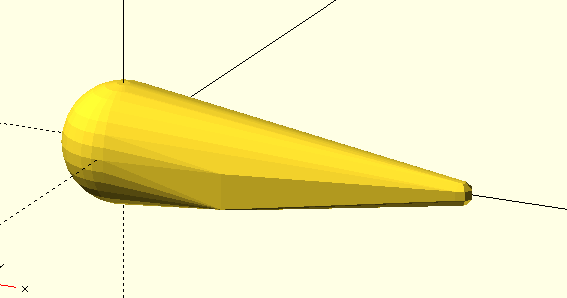Base root code:
```////Basic root Shape

hull() {
translate([60,0,0]) sphere(2);
translate([20,0,0])
rotate([0,180,0]){
cylinder(h = 6, r = 8 );}
sphere(10);
}```

## Random Creation Revision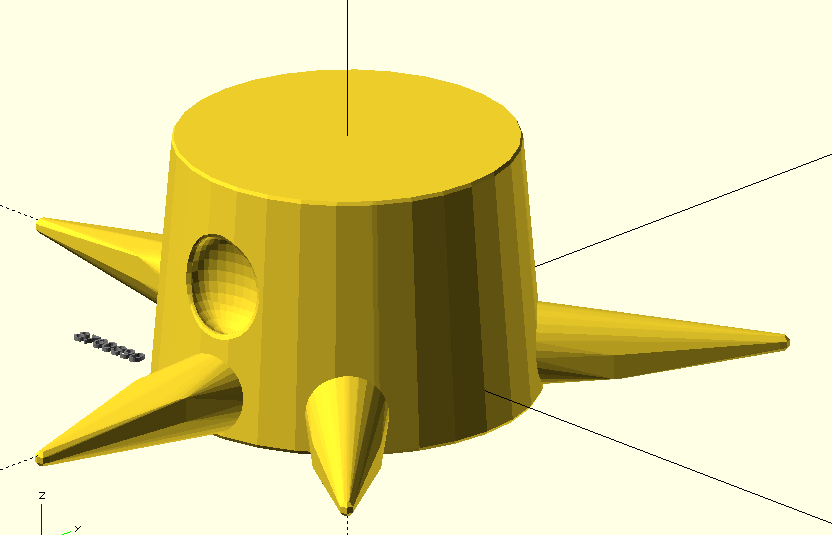I don’t know if this is actually going to be a section or not, however I was having so much fun with this assignment I got a bit carried away and spent an additional 3-4 hours on it working on the roots and revising the previous code. As it currently stands my stump has the random existence of 8 roots and the one hole. I also added a minkowski to the original stump to improve the overall look of the stump.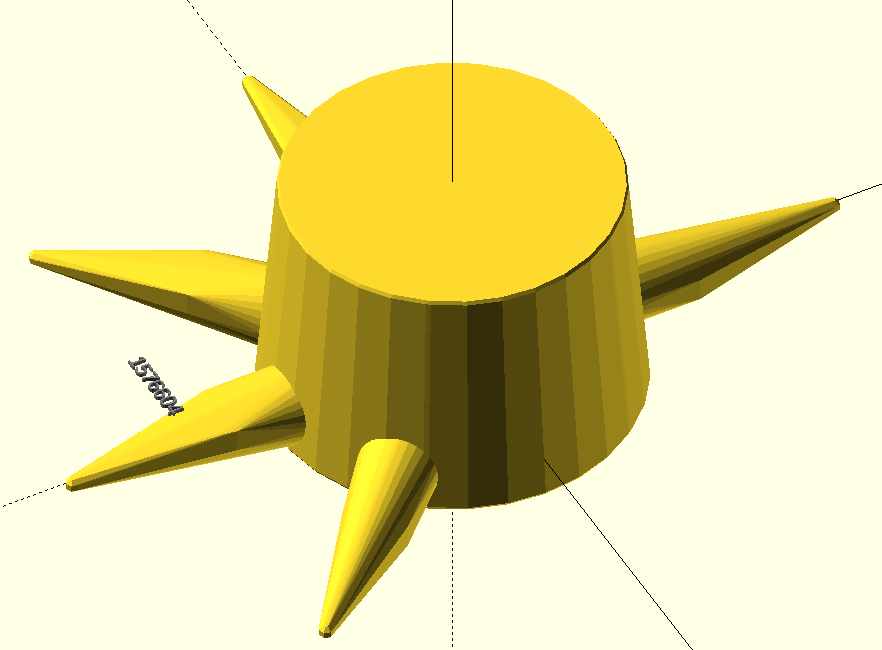Here is the code:

```/////Goals for stump: Variable height or radius, varrying existance of roots/ hole on side

///test values
changing_height = 60;

////Stump

minkowski() {
difference() {
translate([0, 0, 20]){
cylinder(h = changing_height , r1 = 45, r2 = 40,    center = true);}
for (i=[0:10-1]){
if (subrandom3==true) //stump hole existing?
translate([0, -40, 25])
resize(newsize=[24,16,26]) sphere(r=10); ////This is the hole shape!
}
}
sphere(1);
}

/////Roots!!!

if (subrandom3==true)
translate([40,0,-1]){
hull() {
translate([60,0,0]) sphere(2);
translate([20,0,0])
rotate([0,180,0]){
cylinder(h = 6, r = 8 );}
sphere(10);
}
}

if (subrandom3==true)
translate([-40,0,-1])
{rotate([0,180,0]){
hull() {
translate([60,0,0]) sphere(2);
translate([20,0,0])
rotate([0,180,0]){
cylinder(h = 6, r = 8 );}
sphere(10);
}
}
}

if (subrandom3==true)
translate([0,40,-1])
{rotate([0,0,90]){
hull() {
translate([60,0,0]) sphere(2);
translate([20,0,0])
rotate([0,180,0]){
cylinder(h = 6, r = 8 );}
sphere(10);
}
}
}

if (subrandom3==true)
translate([0,-40,-1])
{rotate([0,180,90]){
hull() {
translate([60,0,0]) sphere(2);
translate([20,0,0])
rotate([0,180,0]){
cylinder(h = 6, r = 8 );}
sphere(10);
}
}
}

if (subrandom3==true)
translate([-29,-29,-1])
{rotate([0,180,45]){
hull() {
translate([60,0,0]) sphere(2);
translate([20,0,0])
rotate([0,180,0]){
cylinder(h = 6, r = 8 );}
sphere(10);
}
}
}

if (subrandom3==true)
translate([29,29,-1])
{rotate([0,0,45]){
hull() {
translate([60,0,0]) sphere(2);
translate([20,0,0])
rotate([0,180,0]){
cylinder(h = 6, r = 8 );}
sphere(10);
}
}
}

if (subrandom3==true)
translate([29,-29,-1])
{rotate([0,0,-45]){
hull() {
translate([60,0,0]) sphere(2);
translate([20,0,0])
rotate([0,180,0]){
cylinder(h = 6, r = 8 );}
sphere(10);
}
}
}

if (subrandom3==true)
translate([-29,29,-1])
{rotate([0,180,-45]){
hull() {
translate([60,0,0]) sphere(2);
translate([20,0,0])
rotate([0,180,0]){
cylinder(h = 6, r = 8 );}
sphere(10);
}
}
}

///////COPY PASTE SEED GENERATION INFO/////////////

style1 = 100;

style3 = 5;

// LET OPENSCAD CHOOSE A RANDOM SEED
random_seed = floor(rands(1,9999999,1));

// OR TYPE IN A SEED MANUALLY
// Overrides the slider seed unless set to 0
custom_seed = 0;

// set the random seed
seed = (custom_seed==0) ? random_seed : custom_seed;

// Show random seed? (it won't print even if you say yes)
show_seed = "yes"; //[yes:Yes,no:No]

// Create a string to output the random seed
// thank you laird for showing me how to do this with your code
// from http://www.thingiverse.com/thing:188481
labelString=str(floor(seed/1000000)%10, floor(seed/100000)%10,
floor(seed/10000)%10, floor(seed/1000)%10,
floor(seed/100)%10, floor(seed/10)%10,
floor(seed/1)%10);

// Display the random seed in the render (F5 only)
if(show_seed=="yes"){
translate([0,-80,20])
color("gray")
%linear_extrude(height=1)
text(labelString,size=4,halign="center");
}

// Output the random seed in the log
echo(labelString);

// RANDOM SEQUENCES

// construct the main random sequence
// a list of random real numbers in [0,10] based on the chosen seed
maxSteps=30*1;
random = rands(0,10,maxSteps,seed);
echo(random);

// subsequence of 10 items from the main sequence
// weighted by style1
subrandom1 = [for(i=[0:1-1]) random[i]*style1];
echo(subrandom1);

subrandom3 = [for(i=[0:9-1]) random[i]<=style3];
echo(subrandom3);```

## Final Project with Random Parameters

There hasn’t been a ton of iteration since my last post. This is because I have already accomplished the goals that I wanted at the beginning of the project. This section here is just to fix the varying-height aspect of my design. Unfortunately, even after following the guidelines of the lecture, I am still unable to vary the code.